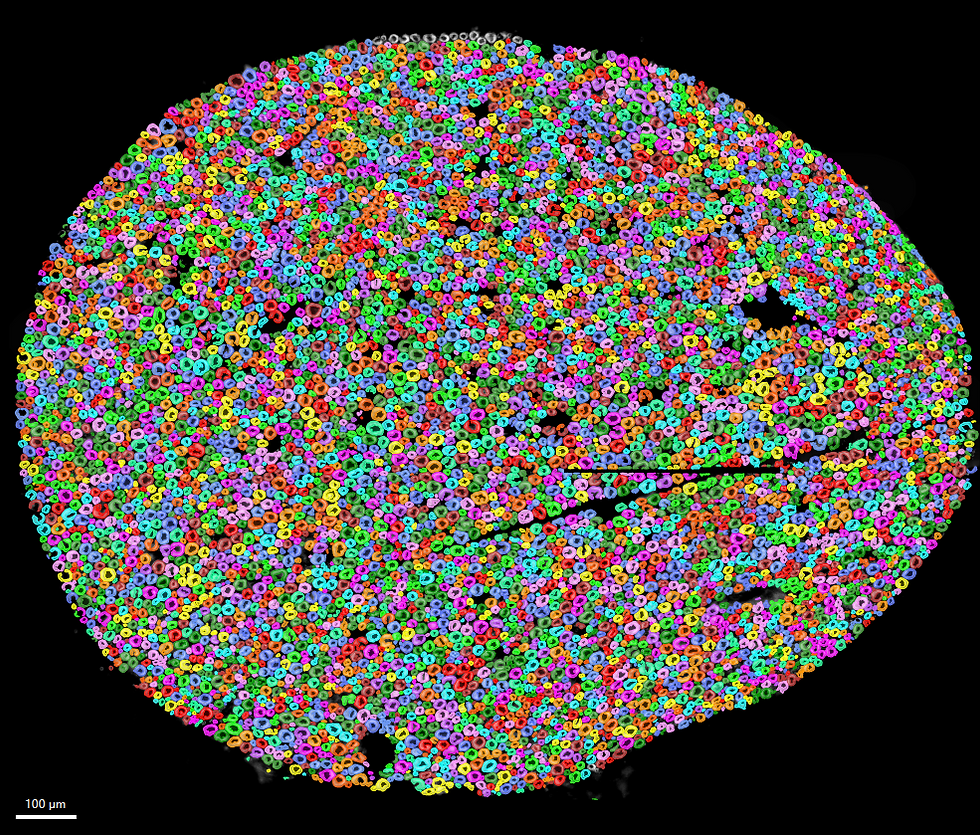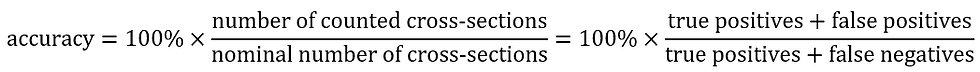top of page
Search

# Precision-Recall Analysis of Peripheral Nerve Myelinated Axon Counting Pipeline

Updated: Nov 3, 2020

Trevor Lancon (DRVISION Technologies, LLC)

Iván Coto-Hernández (Surgical Photonics and Engineering Laboratory, Department of Otolaryngology, Massachusetts Eye and Ear Infirmary and Harvard Medical School)

## Introduction

Microscopy data, as opposed to tabular data, offers a unique perspective for users of machine learning technology: the results can be inspected visually. Egregiously erroneous results are easily rejected as false. In dense datasets, however, it makes sense to employ statistical analysis to inspect whether results agree with reality. In this application, we perform a precision-recall (PR) analysis to quantify the accuracy of a workflow to count cross-sections of myelinated axons in fluorescence micrographs as shown in Figure 1 .Figure 1. Stain-free micrograph of myelinated axons. Each ring represents one cross-section of one axon. Axons greatly range in size. Scale bar is 100 µm.

Receiver operator characteristic (ROC) curves and PR curves elicit similar information when evaluating classification algorithms. However, ROC curves are criticized for reporting optimistic results in cases where there is a large class imbalance within the results; whereas PR curves are more robust in this respect . For this analysis we see results where the true positives count is over 100, whereas false positives and false negatives are less than 20. Thus, we choose to calculate a PR curve as opposed to an ROC curve. ROC curves also require a count of true negatives, which is not easily obtainable from a voxel-based segmentation mask. Furthermore, precision and recall can be broken down into a single F-score for each data point, making our decision-making process easier.

An overview of precision, recall, and the F-score are presented here in brief, but more information can be found at .

Precision is the ability of our algorithm to count only the relevant nerves:Recall is the ability of our algorithm to count all of the relevant nerves (i.e. sensitivity):The F-score is the harmonic mean of precision and recall:## Methods

Image Analysis (Aivia)

Aivia is used to count cross-sections of neurons using a combination of machine learning pixel classification and thresholding. The workflow is as follows:

1. The user trains a random forest machine learning pixel classifier by painting examples of axon and non-axon space on the image. Note that this process is interactive, allowing the user to repaint in multiple iterations using a fast preview of results from a small random forest of shallow decision trees. The user also has some control over which features to use for training, and what kernel sizes to use.

2. Once the preview image looks satisfactory, the user trains the model, then applies it. The applied model is composed of a more complex random forest than preview.

3. The output of the model is a confidence image. Each pixel in this image has a value of 0-255 representing 0-100% confidence, respectively. Good training of the pixel classifier will result in a much higher SNR for the confidence image, and background shading artifacts will also be eliminated.

4. The user then provides two thresholds for the confidence image: the lowest confidence they expect separates neuron from background, and the lowest cross-sectional area allowed for neurons in the results.

Three confidence thresholds for segmentation were chosen to represent 70%, 80%, and 90% confidence (rounded to the nearest integer). Segmentation was performed for each of these thresholds (178, 204, and 230, respectively). Results from all three thresholds are compared here.

The smallest cross-section by area allowed in the results was 300 µm^2.

Results are segmented cross-sections of axons overlaid on the image that the user can inspect for accuracy. The threshold can be re-chosen if necessary.

Precision-Recall and F1 Calculation (napari and Python)

Five regions of interest (ROIs) were manually drawn on the raw image using Aivia’s Annotation Tool as shown in Figure 2. To control the ROI areas and keep them as consistent as possible, a constant zoom (500%) was used in the Aivia 2D viewer. The ROIs have irregular boundaries to fit the non-rectangular shapes of the nerves. Some axons would have intersected the edge of rectangular ROIs and the user would have had to make decisions about whether to include partial axons in their count, introducing an unnecessary source of potential error. The five ROIs were drawn in the center of the image as well as in all four quadrants to account for illumination discrepancies in both X and Y.

Four screenshots were saved of each ROI:

• The raw data with the ROI outline overlaid

• Raw data overlaid with segmentation from 70%

• Raw data overlaid with segmentation from 80%

• Raw data overlaid with segmentation from 90%

Each screenshot was then passed to a custom Python function to load the image in napari . This allowed tallying of true positives, false positives, and false negatives in the segmentations. This function can be found on Github at . For our purposes, the following definitions are used for these classes:

• True positives are correctly segmented cross-sections

• False positives are any objects that are included in the segmentation that do not represent true, full axon cross-sections

• False negatives are any nerve cross-sections that are missed, and therefore not included in the segmentation

To clarify how decisions are made in cases of over-/under-segmentation:

• For cross-sections that are split by weak points in the confidence map, we count the larger (as judged by eye) as a true positive, and all other components of that same nerve that are segmented as false positives.

• For over-segmented cross-sections that are erroneously conjoined with their neighbors, we count the most prominent cross-section as a true positive, and all other cross-sections within that over-segmentation as false negatives.

The count automatically returned by Aivia is equal to the sum of all true positives and false positives.

The nominal count is equal to the sum of all true positives and false negatives.Figure 2. Five irregular ROIs are shown in blue. ROIs are referred to as A - E from top-left to bottom-right.

## Results and Conclusions

Figures 3 – 5 show segmentations of the entire micrograph for confidence thresholds of 70%, 80%, and 90%, respectively. Note how qualitatively evaluating the accuracy of the algorithm’s performance on such a large field of view (FOV) with comparatively small objects is practically impossible.Figure 3. Segmentation results for a confidence threshold of 70% (corresponding to an intensity threshold of 178/255).Figure 4. Segmentation results for a confidence threshold of 80% (corresponding to an intensity threshold of 204/255).Figure 5. Segmentation results for a confidence threshold of 90% (corresponding to an intensity threshold of 230/255).

Counts are shown for each confidence threshold and each ROI in Table 1, along with precision, recall, and F-scores.Table 1. Results from counting all ROIs and calculation of precision, recall, and F1 from all confidence thresholds. True positives (TP), false positives (FP), and false negatives (FN) are all shown.

An example of counting results is also shown in Figure 6, where we compare the raw image with ROI A overlaid with the segmentation results from the 70% confidence threshold, as well as the points layers added using napari. Images such as these were saved for each ROI and each threshold but are excluded here for brevity.Figure 6. Montage showing the raw images overlaid with ROI A (top), the segmentation results from a confidence threshold of 70% (middle), and segmentation results with points layers from napari overlaid (bottom). In the points layers, green dots indicate true positives, yellow dots indicate false negatives, and red dots indicate false positives.

Plotting the data from Table 1, we can construct a PR curve as shown in Figure 7. Precision and recall were both relatively high for all datasets, so a zoomed version of the plot is also shown.Figure 7. PR curves for all ROIs and thresholds. The image on the right shows the same plot with zoomed axes (indicated by dotted square on the left). Thresholds of 178, 204, and 230 corresponding to 70%, 80%, and 90% thresholds of the confidence image returned by the machine learning pixel classifier, respectively.

Some immediate conclusions that seem clear from the PR curve in Figure 7 are:

• All thresholds perform relatively well.

• The 90% confidence threshold (230) is clearly not as precise as either the 70% or 80% threshold.

• The 90% confidence threshold (230) sensitivity appears to be less, but not significantly so.

• Since the 70% and 80% thresholds are so similar, it makes sense to break them down to a simpler decision by observing the F1.

If we look at the F1 for each ROI after filtering out the 90% threshold as shown in Figure 8, we see that choosing a threshold of 70% maximizes the tradeoff between precision and recall for all ROIs except C, where the F1 is slightly higher (0.000385).Figure 8. Comparison of F scores for confidence thresholds of 178 and 204, corresponding to 70% and 80%, thresholds of the confidence image returned by the machine learning pixel classifier, respectively.

Thus, for our case, it makes sense to choose the 70% confidence threshold to segment nerve cross-sections. This will maximize our tradeoff between how precise and how sensitive this trained model is for counting axons in nerve cross-sections.

Finally, we are unable to calculate accuracy of this threshold based on its traditional definition due to not being able to count true negatives. We can define our own estimate of accuracy, however, as follows:Using only the TP, FP, and FN rates from the 70% confidence threshold, this accuracy estimate is 92.468%.

In conclusion, we see that choosing a confidence threshold of 70% for segmenting nerve cross-sections following the application of a properly trained machine learning pixel to this type of data results in a cross-section counting accuracy of approximately 92%. The precision-recall analysis suggests that Aivia could be employed for rapid quantification of myelinated axon counts within peripheral nerve sections to aid surgical decision-making in nerve transfer procedures .

You can see an in-depth account of the sample preparation and image acquisition workflow in A Rapid Protocol for Intraoperative Assessment of Peripheral Nerve Myelinated Axon Count and its Application to Cross-Facial Nerve Grafting from the Surgical Photonics and Engineering Laboratory at Harvard Medical School .

## References

1. W Wang, S Kang, I. Coto Hernández and N Jowett, “A Rapid Protocol for Intraoperative Assessment of Peripheral Nerve Myelinated Axon Count and its Application to Cross-Facial Nerve Grafting,” Plast Reconstr Surg 143(3):771-778 (2019)

2. Davis, J and Goadrich, M. The Relationship Between Precision-Recall and ROC Curves. Proc. of the 23rd Int. Conf. on Machine Learning

3. Koehrsen, W. Beyond Accuracy: Precision and Recall. Towards Data Science Blog

4. napari: multi-dimensional image viewer for Python

5. napari_count_tfpn.py

6. Hernández, I.C., Yang, W., Mohan, S. and Jowett, N. (2020), Label‐free Histomorphometry of Peripheral Nerve by Stimulated Raman Spectroscopy. Muscle Nerve

See All
bottom of page